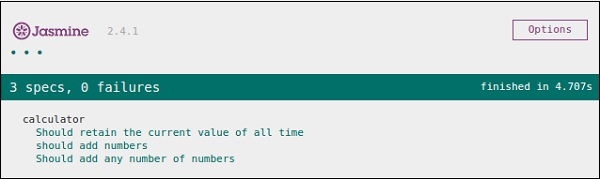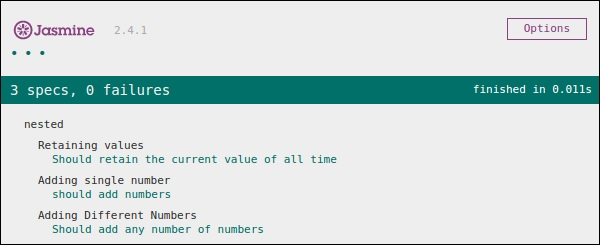# JasmineJS - Building Blocks of Test

In this chapter, we will discuss the building blocks of test by Jasmine.

## Suite Block

Jasmine is a testing framework for JavaScript. Suite is the basic building block of Jasmine framework. The collection of similar type test cases written for a specific file or function is known as one suite. It contains two other blocks, one is “Describe()” and another one is “It()”.

One Suite block can have only two parameters, one “name of that suite” and another “Function declaration” that actually makes a call to our unit functionality that is to be tested.

In the following example, we will create a suite that will unit test add function in add.js file. In this example, we have our JS file named “calculator.js” which will be tested through Jasmine, and the corresponding Jasmine spec file is “CalCulatorSpec.js”.

### Calculator.js

```window.Calculator = {

currentVal:0,
varAfterEachExmaple:0,

this.currentVal += num1;
return this.currentVal;
},

var sum = this.currentVal;

for(var i = 0; i < arguments.length; i++) {
sum += arguments[i];
}

this.currentVal = sum;
Return  this.currentVal;
},
};
```

### CalCulatorSpec.js

```describe("calculator",function() {

//test case: 1
it("Should retain the current value of all time", function () {
expect(Calculator.currentVal).toBeDefined();
expect(Calculator.currentVal).toEqual(0);
});

//test case: 2
});

//test case :3
it("Should add any number of numbers",function () {
});
});
```

In the above function, we have declared two functions. Function add will add two numbers given as an argument to that function and another function addAny should add any numbers given as an argument.

After creating this file, we need to add this file in “SpecRunner.html” inside the head section. On successful compilation, this will generate the following output as a result.## Nested Suites Block

Suite block can have many suite blocks inside another suite block. The following example will show you how we can create a different suite block inside another suite block. We will create two JavaScript files, one named as “NestedSpec.js” and another named as “nested.js”.

### NestedSpec.js

```describe("nested",function() {

// Starting of first suite block
// First block

describe("Retaining values ",function () {

//test case:1
it ("Should retain the current value of all time", function () {
expect(nested.currentVal).toBeDefined();
expect(nested.currentVal).toEqual(0);
});
}); //end of the suite block

//second suite block
describe("Adding single number ",function () {

//test case:2
});
}); //end of the suite block

//third suite block

//test case:3
it("Should add any number of numbers",function() {
});
}); //end of the suite block
});
```

### Nested.js

```window.nested = {

currentVal: 0,

this.currentVal += num1;
return this.currentVal;
},

Var sum = this.currentVal;

for(var i = 0;i < arguments.length; i++) {
sum += arguments[i];
}

this.currentVal = sum;
return this.currentVal;
}
};
```

The above piece of code will generate the following output as a result of running specRunner.html file after adding this file inside the head section.## Describe Block

As discussed earlier describe block is a part of Suite block. Like Suite block, it contains two parameters, one “the name of the describe block” and another “function declaration”. In our upcoming examples, we will go through many describe blocks to understand the working flow of Jasmine suite block. Following is an example of a complete describe block.

```describe("Adding single number ",function () {

});
}
```

## IT Block

Like describe block we have been introduced to IT block too. It goes within a describe block. This is the block which actually contains each unit test case. In the following code, there are pieces of IT block inside one describe block.

```describe("Adding single number ",function () {

// test case : 1
});

//test case : 2
});
}
```

## Expect Block

Jasmine Expect allows you to write your expectation from the required function or JavaScript file. It comes under IT block. One IT block can have more than one Expect block.

Following is an example of Expect block. This expect block provides a wide variety of methods to unit test your JavaScript function or JavaScript file. Each of the Expect block is also known as a matcher. There are two different types of matchers, one inbuilt matcher and another user defined matchers.

```describe("Adding single number ",function () {

// test case : 1